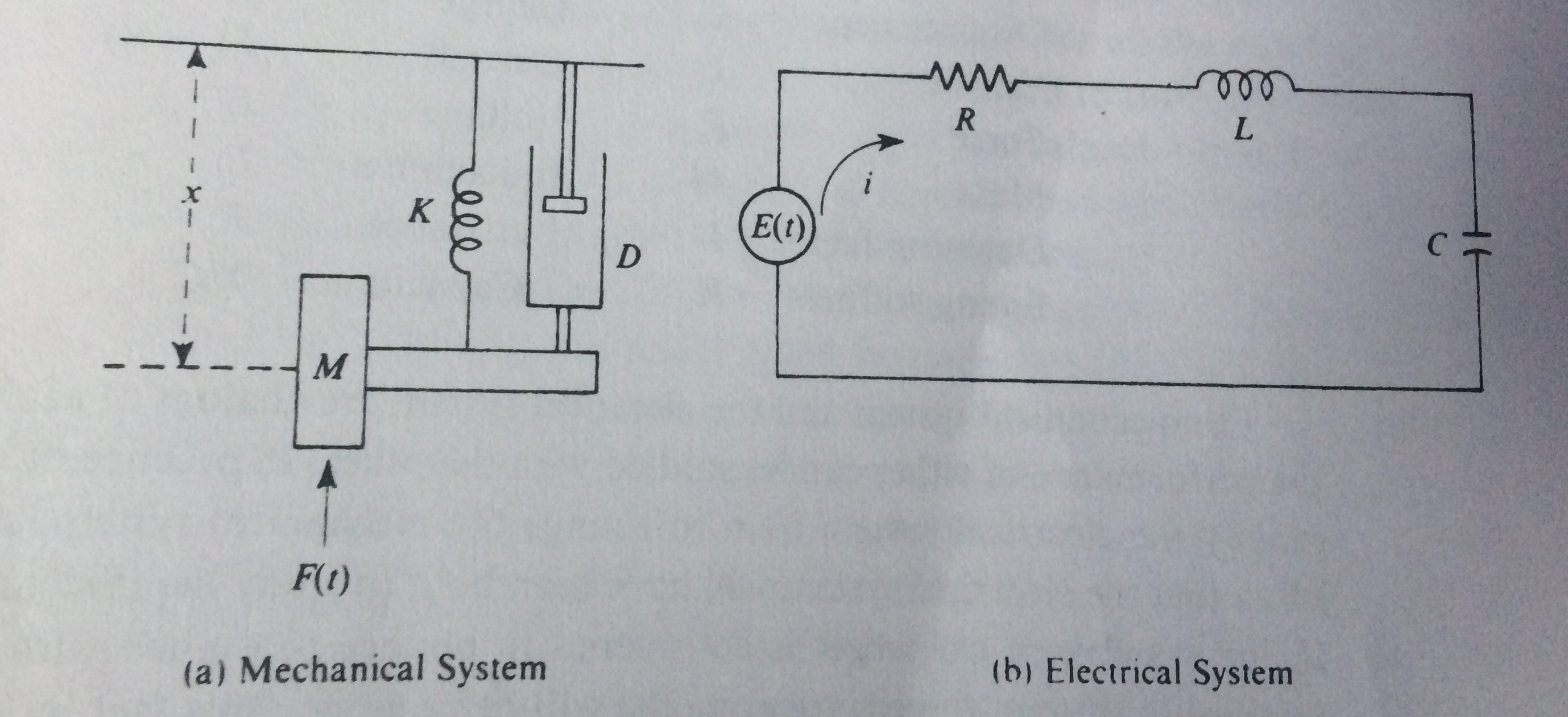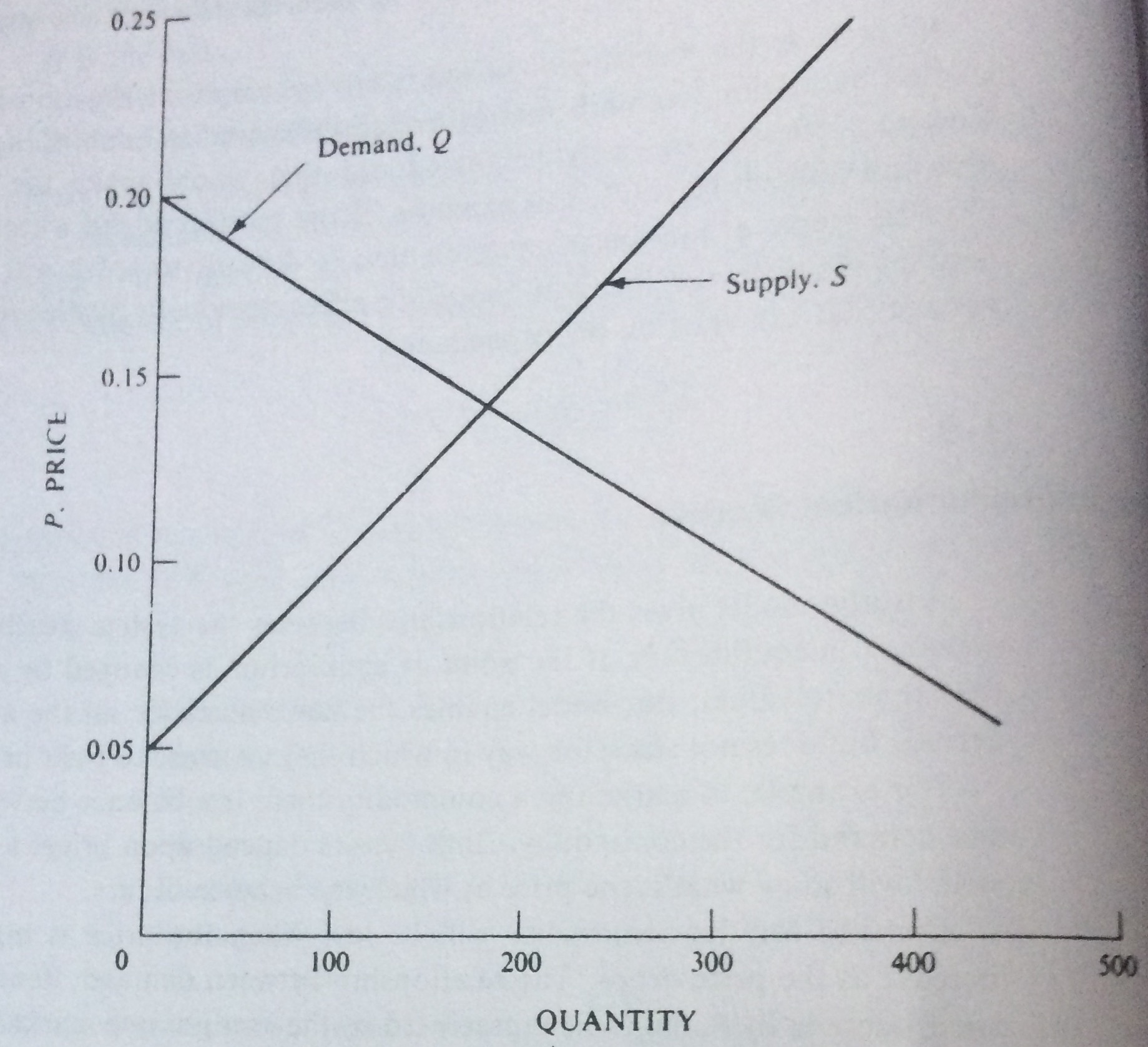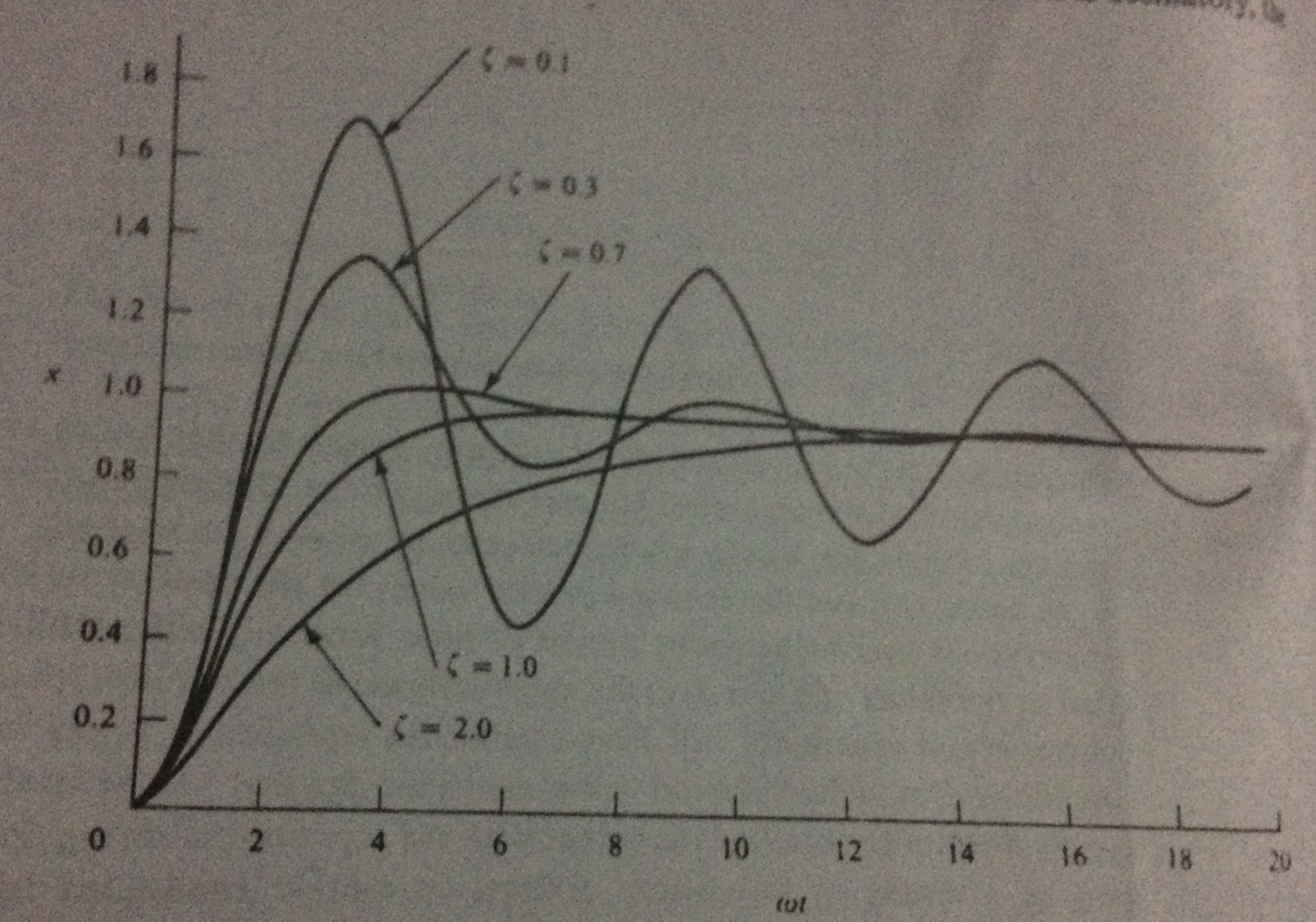### Notes of Simulation and Modelling [CT 753]

#### Physical and Mathematical models

Physical Model

- Physical model is the smaller or larger physical copy of an object being modeled.
- The geometry of model is just the rescaled view of the object it represents.
- The horizontal and vertical scaling may not be balanced as per the requirements of the model.
- The physical model helps in visualization of the object taken into consideration in an effective way.
- It is also used to solve equations with the particular boundary conditions.

Static Physical Model:

- Static physical model is the physical model which describes relationships that do not change with respect to time.
- Such models only depict the object’s characteristics at any instance of time, considering that the object’s property will not change over time.
- Eg : An architectural model of a house, scale model of a ship and so on.

Dynamic Physical Model:

- Dynamic physical model is the physical model which describes the time varying relationships of the object properties.
- Such models describes the characteristics of the object that changes over time.
- It rely upon the analogy between the system being studied and some other system of a different nature, but have similarity on forces that directs the behavior of the both systems.
- Eg: A model of wind tunnel, a model of automobile suspension and so on.

Mathematical Model

- Mathematical model is the model which is composed of a symbols and logic.
- Such model is also called an abstract model.
- It describes the system using mathematical concepts.
- The mathematical model is used to explain the system and to study the effects of different components, and to make predictions about the behavior of the system.
- It generally consists of following major elements:
a) Governing equations
b) Defining equations
c) Constitutive equations
d) Constraints

Static Mathematical Model:

- Static mathematical model is the mathematical model that represents the logical view of the system in equilibrium state.
- Such models are time-invariant.
- It is generally represented by the basic algebraic equations.
- Eg: An equation relating the length and weight on each side of a playground variation, supply and demand relationship model of a market and so on.

Dynamic Mathematical Model:

- Dynamic mathematical model is the mathematical model that accounts for the time dependent changes in the logical state of the system.
- Such models are time-variant.
- It is generally represented by differential equations or difference equations.
- Eg: The equation of motion of planets around the sun in the solar system.

Examples: Model

Scale Model of a Ship (Static Physical Model):

- A scale model of a ship is a simplified approach to determine the exact measurements of the components of the ship during its construction.
- It removes the necessity of drawing complex 3D shapes to just analyze the ship model and their measurements.
- The measurements on scale model are later converted into the values that would occur in the real system.

Automobile Suspension Model (Dynamic Physical Model):

- Consider the mechanical system in which a mass is subjected too an applied force F(t) that varies with time, a spring whose force is proportional to extension or contraction and a shock absorber that exerts a damping force proportional to the velocity of the mass.
- This represent the suspension of an automobile wheel when the automobile is assumed to be immobile in a vertical direction.
- The problem can be defined by the differential equation:
Mx’’ + Dx’ + Kx = KF(t) → 1
where, M = mass, K = stiffness of the spring and D = damping factor of shock absorber.
- Analyzing this system is very much difficult and expensive. So, it can be modeled with the electrical system as shown:
- The electrical circuit consists of an inductance L, a resistance R and a capacitance C, connected in series with a voltage source that varies with time according to function E(t). If q is the charge in capacitance, it can be defined as:
Lq’’ + Rq’ + q/C = E(t)/C → 2
- Equation 1 and 2 are similar in behavior, so the mechanical system can be simulated using an electrical system with more efficiency and with low cost.
- The equivalence between the two systems are:
a) Displacement x = Charge q
b) Velocity x’ = Current I, q’
c) Force F = Voltage E
d) Mass M = Inductance L
e) Damping Factor D = Resistance R
f) Spring stiffness K = Inverse of Capacitance 1/C
g) Acceleration x’’ = Rate of change of current q’’Market Model – Supply and Demand (Static Mathematical Model):

- Consider a market model assuming the linear behavior of supply and demand with price.
- Demand for a product will be low when the price is high and will be high when the price is low.
- Supply for a product will be high when the price is high and will be low when the price is low.
- If conditions remain stable, the price will settle to the point at which demand equals supply.
- The relations can be stated mathematically as:
Q = a – bP
S = c + dP
S = Q
where, S = supply, Q = demand and P = price
- The linear market model is shown below:Automobile Suspension (Dynamic Mathematical Model):

- The automobile suspension equation is written as:
x’’ + 2dw x’ + w^2 x = w^2 F(t)
where 2dw = D/M and w^2 = K/M; d = damping ratio, w = frequency of oscillation
- The solution for several values of d in terms of the variable wt is shown below:
- When d is less than 1, the motion is oscillatory and w = 2 * pi * f
- The relationship guides to select the spring and shock absorber to get that type of motion.